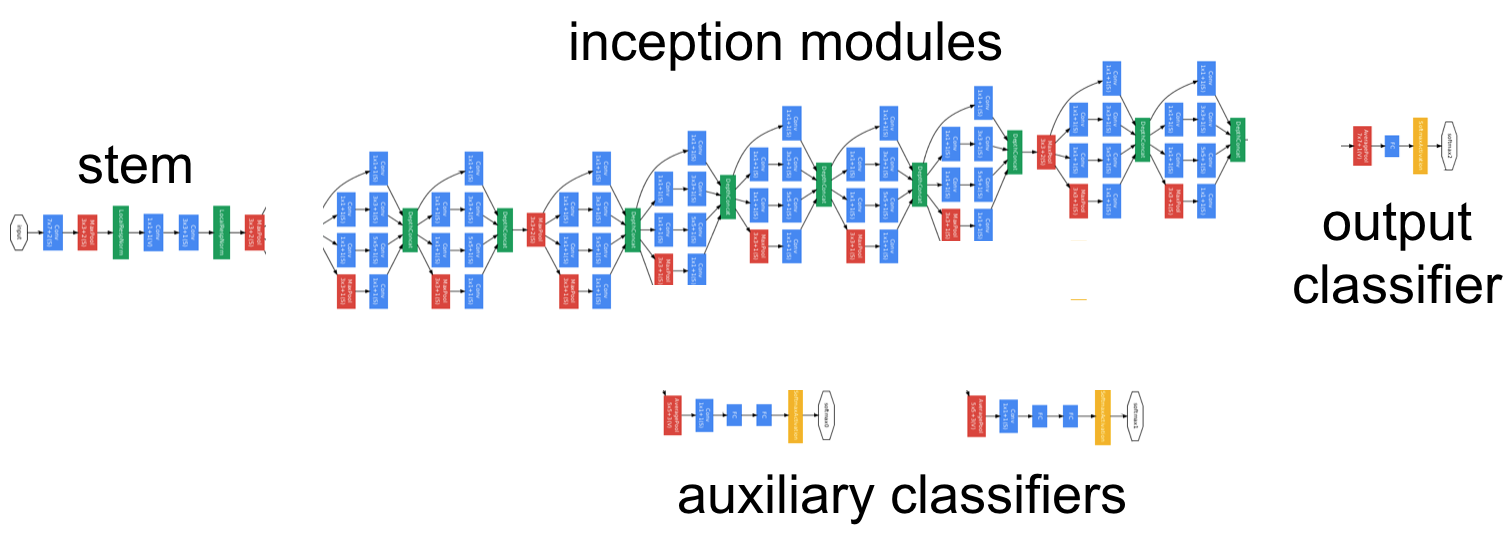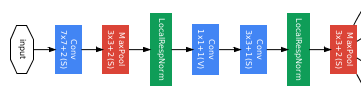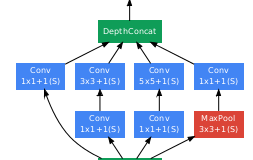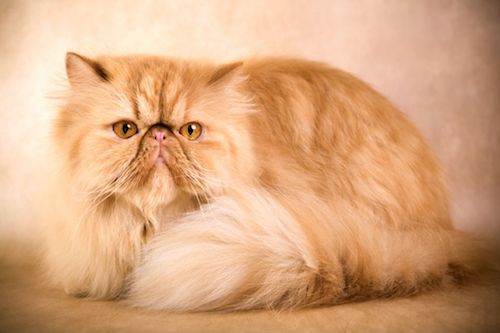### googlenet in keras

In this new era of deep learning, a number of software libraries have cropped up, each promising users speed, ease of use, and compatibility with state-of-the-art models and techniques. The go-to library in the Caltech vision lab has been Caffe, an open-source library developed by Yangquing Jia and maintained by the Berkeley Vision and Learning Center (BVLC). It has been the gold standard in terms of speed and offering the latest pre-trained models (AlexNet, GoogLeNet, etc.). However, for trying anything other than what's already been done, Caffe can be rigid and difficult to adapt. Diving into the C++ code to implement a new layer can be a daunting task, and don't get me started on those .prototxt files! For research, I need a deep learning library that I can easily adapt to whatever experiment I'm working on, so I went searching for greener pastures and found Keras, a python library developed by François Chollet that runs on top of Theano and Tensorflow. Installing Keras and either of these backend libraries is fairly easy (just pip install), and Keras itself achieves an excellent balance of simplicity and adaptability.

However, Keras doesn't contain the degree of pre-trained models that come complete with Caffe. There are a number of github repositories by devoted Keras followers hosting implementations of AlexNet, VGG, GoogLeNet, etc., but from what I could tell, these models didn't exactly correspond to the models I had worked with in Caffe. As I often work with GoogLeNet (which is also referred to as Inception V1), I took it upon myself to transfer the weights from Caffe into an exact replica in Keras. The following is a walkthrough of my method.

Before we begin, if you're not interested in getting into the details of how to implement GoogLeNet in Keras yourself, feel free to just download the model. Keras models are defined by two files: a json file containing the model architecture and an hdf5 file containing the model's weights. You can use the link below to download a zip folder containing the architecture and weight files for GoogLeNet.

To load the model, run the following:

I've also created a GitHub Gist that contains a script with the entire model definition.

I've laid this blog post out in two sections, constructing the network architecture and transferring the weights, however, these two phases went hand in hand. Along the way, I used the weights to generate activations to verify the network architecture.

Constructing the Network ArchitectureThe behemoth that sits above is GoogLeNet. As part of an ensemble of other similar models trained by the researchers at Google, GoogLeNet achieved a top-5 error rate of 6.67% on the 2014 ImageNet classification challenge. What that means is this: if you have an image of an object that is contained in the 1,000 object classes of the ImageNet dataset (all sorts of animals, household objects, vehicles, etc.), 93.33% of the time the correct object class will be contained in the GoogLeNet ensemble's top five predictions. Considering that ImageNet consists of many fine-grained object categories and that some images contain multiple object categories, this is an incredible feat, nearly on par with human performance. While at first glance the model may appear incredibly complex, upon closer inspection, the overall structure of the model can be broken down into a few basic sections: the stem, the inception modules, the auxiliary classifiers, and finally the output classifier.GoogLeNet starts with a sequential chain of convolution, pooling, and local response normalization operations, in a similar fashion to previous convolutional neural network models, such as AlexNet. Later papers on the inception architectures refer to this initial segment as the 'stem'. Shown below, the stem stands in contrast to the rest of the GoogLeNet architecture, which is primarily made up of what are referred to as 'inception' modules. The authors cite technical issues for including the stem rather than training a network made up entirely of inception modules. It will be interesting to see whether this stem section remains a part of future networks.The basic building block of GoogLeNet, the inception module, is a set of convolutions and poolings at different scales, each done in parallel, then concatenated together. Along the way, $1 \times 1$ convolutions are used to reduce the dimensionality of inputs to convolutions with larger filter sizes. This approach results in a high performing model with drastically fewer parameters. GoogLeNet, in fact, has a factor of 12 times fewer parameters than AlexNet. Why the name inception, you ask? Because the module represents a network within a network. If you don't get the reference, go watch Christopher Nolan's Inception...computer scientists are hilarious.The above diagram shows an inception module. GoogLeNet contains nine of these modules, sequentially stacked, with two max pooling layers along the way to reduce the spatial dimensions. Due to the depth of this architecture, the authors added two auxiliary classifiers branching from the main network structure. The purpose of these classifiers is to amplify the gradient signal back through the network, attempting to improve the earlier representations of the data. However, with the introduction of batch normalization, these classifiers have been ignored in recent models.Finally, we get to the output classifier, which performs an average pooling operation followed by a softmax activation on a fully connected layer.In total, the network uses the standard operations: convolution, pooling, normalization, and fully-connected layers. Unbeknownst to me, each of these operations are performed differently across different software libraries, so each operation required some hacking to convert from Caffe and Keras.

Let's start by looking at the input. Opening GoogLeNet's deploy.prototxt file from Caffe, we first see

What we have is a network named GoogLeNet that takes a 4-D input blob "data" with input dimensions (10, 3, 224, 224), i.e. batches of 10 images, each with 3 channels (note: in BGR order!), of size 224 $\times$ 224. In Keras, working with the Functional API, this is equivalently written as

The batch size is ommitted for the time being, as it gets set once we train or test with the model. Moving on, let's look at the first convolutional layer in Caffe:

What we see is a convolutional layer named "conv1/7x7_s2" that takes input from "data", applies a set of 64 7 $\times$ 7 convolutional filters with a stride of 2 and a padding of 3, then passes the activations through the ReLU layer "conv1/relu_7x7". In Keras, we can implement this using a Convolution2D layer as follows:

We use the subsample and border_mode keyword arguments to handle the stride and padding respectively. By setting border_mode='same', we tell Keras that we want to pad the input with zeros such that the spatial output size of the layer (if we were to neglect the stride) would be the same as the input size. For a filter size of 7 $\times$ 7, this would correspond to a padding of 3, as in Caffe. Next, we move on to a pooling layer:

This max-pooling layer takes input from "conv1/7x7_s2" and uses a 3 $\times$ 3 window with stride 2 to subsample the maximum activations from the convolution. Since the layer's prototxt definition does not specify any padding, this is considered to be a 'valid' operation, i.e. only take maxima at locations where the window completely overlaps the input. In Keras, we would implement this using a MaxPooling2d layer:

However, this operation is not consistent from Caffe to Keras. After some frustration, I found that Caffe actually does pad the end of both spatial dimensions. In this way, with indexing ranging from (0, 0) to (111, 111), the first pooling location is centered at (1, 1), but the last pooling location is centered at (111, 111). Valid at the beginning, same at the end. My solution: zero-pad the result from the convolutional layer, then use a custom layer to remove the zeros from the beginning of both dimensions (the first row and column). Thus,

where PoolHelper is a custom layer, implemented as

Sure enough, this does the trick. Now on to normalization. In Caffe, the local response normalization layers are defined as

What do the hyperparameters local_size, alpha, and beta mean? In Caffe, the local response normalization is performed for each example by normalizing along the feature (channel) dimension. This normalization is performed only over a small window, the local size, over features at every spatial location. The parameters $\alpha$ and $\beta$ come in through the normalization equation: \begin{equation} LRN(x_{f,r,c}) = \left( k + \frac{\alpha}{n} \sum_{i=f-\frac{n}{2}}^{f+\frac{n}{2}} x_{i,r,c}^2 \right)^\beta. \end{equation} Let's deconstruct this equation. The local response normalization of the input $x_{f,r,c}$ at a particular feature $f$ at row $r$ and column $c$ is given by an offset $k$ plus a scaling coefficient $\alpha$ times the average squared input over the centered window of size $n$ (the local size), all taken to the power of $\beta$. Again, note that the window extends only over the feature dimension, meaning that each spatial location is normalized separately. In Caffe, $k$ is set to 1.

In the latest version of Keras, the only form of normalization is the BatchNormalization layer. And while this layer is useful and versatile, especially with the widespread adoption and nice theoretical motivations of batch normalization, it does not perform local response normalization in the same way as Caffe. Previous versions of Keras included a LRN2D layer, which was adapted from pylearn2, however I had to make modifications to this code to obtain the identical operation performed by Caffe. The layer definition is below:

I have added comments to explain the steps along the way. Now we can add the LRN layer to our network:

Now you might be thinking to yourself, "We're only three layers in! This is going to take forever!" Not to fear, after accounting for the differences in pooling and normalization between Caffe and Keras, the rest of the network is a straightforward conversion. The rest of the stem can be completed similarly to the first three layers. I'll spare you the prototxt version, but in Keras, the first inception module looks like this:

We see 1 $\times$ 1, 3 $\times$ 3, and 5 $\times$ 5 convolutions with varying numbers of filters in addition to a pooling layer. At the end, the outputs of these different pathways are concatenated using Keras' merge layer. The next inception module will then take its input from this concatenated layer. The rest of the inception layers are exactly identical, but with different numbers of filters.

Finally, we get to the classifiers. Since the auxiliary classifiers are not included in Caffe's deploy model, I will focus on the final classifier (the auxiliary classifiers are nearly identical). At the beginning of each classifier branch is an average pooling layer. In Caffe, this is written as

In Keras, we can use the AveragePooling2D layer to implement this:

We then come to a dropout layer.

This is simply implemented using Keras' Dropout layer. However, before this point, I flatten the input to get rid of the spatial dimensions, which by this point, are both of size 1.

...and finally the output softmax layer.

This is implemented in Keras using a Dense layer (with default linear activation) followed by a softmax Activation layer.

Finally, in Keras, we need to turn the set of layers into a model by specifying the input and output.

And that's all there is to it! At this point, to train the model, we would simply need to compile the it (likely using 'categorical_crossentropy' for the loss function) and start feeding in batches of training examples. Don't forget: in order to train, you will also want to add the W_regularizer argument to each of the convolution and fully-connected layers. Caffe specifies the regularization hyperparameter as being 0.0002.

However, the main point of reconstructing this network in Keras was to take advantage of the pre-trained weights from Caffe. In the next section, I'll walk through the process of transferring these weights over.

Transferring the Weights

GoogLeNet's weights are contained in a caffemodel file and can be accessed by loading them in Caffe. With the deploy.prototxt file, this is done as follows:

The model's weights and biases are accessed through net.params, whereas each layer's activations are accessed through net.blobs. Therefore, to copy over the weights, we can run the following script:

We run through all of the layers, copying over the weights and biases from Caffe, then set those parameters in the corresponding layer in Keras. Unfortunately, if we run this script, we will encounter two errors, one of which will be obvious, and the other one not. The first error occurs due to the fact that the weights for fully-connected layers are transposed between Caffe and Keras. This is simply a convention between libraries. We can remedy this error by adding the following:

The other error is more difficult to catch. Caffe and Keras do not implement convolutional layers in the same manner. Keras (really Theano) performs convolution, whereas Caffe performs correlation. For more information on how these differ, see this explanation. In real terms, this simply means that we need to rotate each of the filters by 180$^{\circ}$:

Upon adding these two catches into our copying script, we can now copy the weights over. And there you have it, GoogLeNet in Keras!

Testing the Model

Just to make sure everything went off without a hitch, let's try running a sample image through both networks to verify that the activations are consistent. While copying over the weights, this was how I debugged the Keras model. Let's use the sample image used by Caffe, that adorable tabby cat kitten:First we'll preprocess the image by subtracting the channels means, changing the channel ordering, switching the spatial and channel dimensions, cropping the image, and adding an extra dimension for the batch.

Now we can run the image through Caffe. We reshape the data layer to contain a single example and pass our image into this layer. We then call the network's forward function to propagate the activations through the layers.

To get the activations in Keras, we will define a function that takes in a model, a layer, and an input and returns that layer's activations.

We can now compare activations between the two networks at any layer. If we have a layer called layer_name, we can get the activations as:

Rather than show the activations for each and every layer, I will show the final output of each model by setting layer_name='prob'. In Caffe, the image of the kitten results in

n02123394, Persian cat, 0.79362965

n02127052, lynx, catamount, 0.081304103

n02123159, tiger cat, 0.074268937

n02123045, tabby, tabby cat, 0.029699767

n04589890, window screen, 0.0024248438

While in Keras, we get the following:

n02123394, Persian cat, 0.79363048

n02127052, lynx, catamount, 0.081303641

n02123159, tiger cat, 0.074268863

n02123045, tabby, tabby cat, 0.029699543

n04589890, window screen, 0.0024248327

Immediately, we see that the network struggled with this image. Honestly...Persian cat?I'm no cat expert, but the original image looks to be a tabby. I guess object classification (and computer vision more generally) hasn't been solved. Comparing the probabilities between the two networks, we see that they match up to about four decimal places. I'm not sure specifically where this comes from, but it could be an artifact of the number of bits used to store activations throughout the networks. For all intents and purposes, the networks produce identical outputs. Now get out there and have some fun with GoogLeNet!